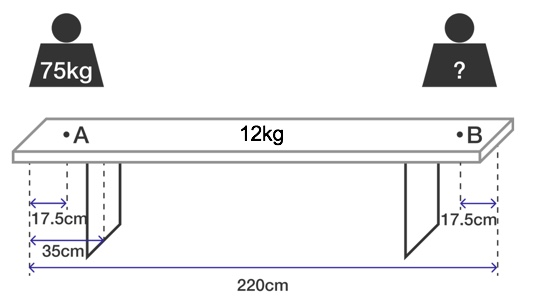# Bierbank

Sitting down at the end of an empty ale-bench is usually a bad idea because the bank could overturn immediately. In the figure below, what is the weight needed on point $B$ just enough to compensate a body weight of $75\text{ kg}$ on point $A?$Details and Assumptions:

• The mass of the wooden panel is $m = 12 \,\text{kg}.$
• The length of the bench is $l = 2.2 \, \text{m}$ and its overhang is $35\,\text{cm}$ on each side.
×

Problem Loading...

Note Loading...

Set Loading...